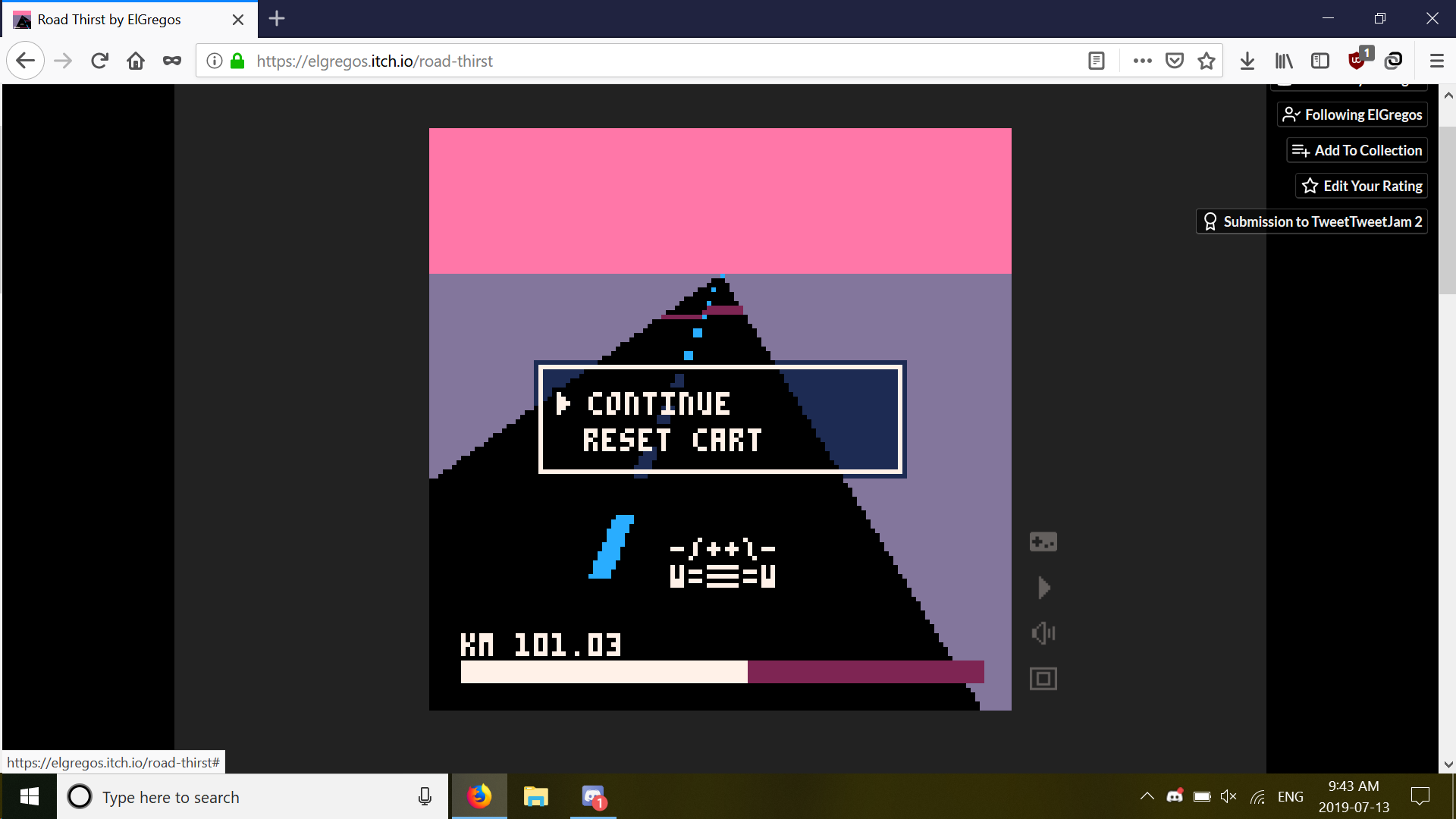Made in 557 chars of Pico-8 for TweetTweetJam 2.

This is a nice car, only problem is its small tank. But that dirty road will luckily provide all the gas you need. Don't pick too much though or your tank will explode! How far will you go?

Use any button to switch lane. This means using left & right keys works too.

Here's the complete code, just copy & paste in Pico-8 to get the whole game:

```srand(3)d=.1t=0l=.5g=0r=rectfill
h={}for n=0,999 do
function _update60()
cls(14)x=64w=0k=20
for n=0,1,1/95 do
j=flr(k+t)y=n*96+32
r(0,y,127,y,13)for m=0,1 do
r(x-w+w*m,y,x+w*m,y,h[j%(#h/2)*2+m+1])end
if(j%2>0)r(x-w*.03,y,x+w*.03,y,12)
w+=1
x+=l
k*=.96
end
if(pget(64,95)==2 and not q)g+=1
w=t%1<.5 and"m"or"w"
?"-/++\\-\n"..w.."=▤="..w,53,90,7
r(7,117,121,121,2)i=7
if(g<47 or g>99)i=8
if(q)i=g%2+7
r(7,117,g,121,i)
?"km "..flr(t)/100,7,111,7
q=q or g>121
g=mid(7,g-.1,121)
t+=.008*min(g-7,40)
if(btnp()>0)d=-d
l=mid(-.5,l+d,.5)end```
 Status Released Platforms Windows, macOS, Linux, HTML5 Rating Rated 5.0 out of 5 stars(3) Author ElGregos Genre Survival, Racing Made with PICO-8 Tags 8-Bit, Abstract, Arcade, PICO-8